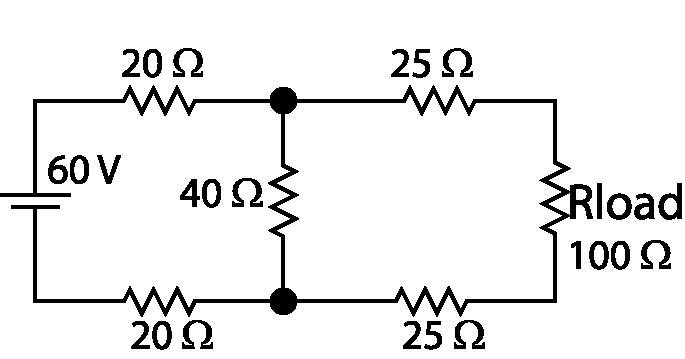# Simple Circuit Problem

• Engineering
I don't under stand the question

"Determine the equivalent Thevenin voltage source external to the load resistor"

here's a diagram of the circuit. All I need to know is where on the circuit I'm suppose to calculate this, then I can take it from there. Do I take out the load resistor from the circuit and find the voltage?

Diagram: